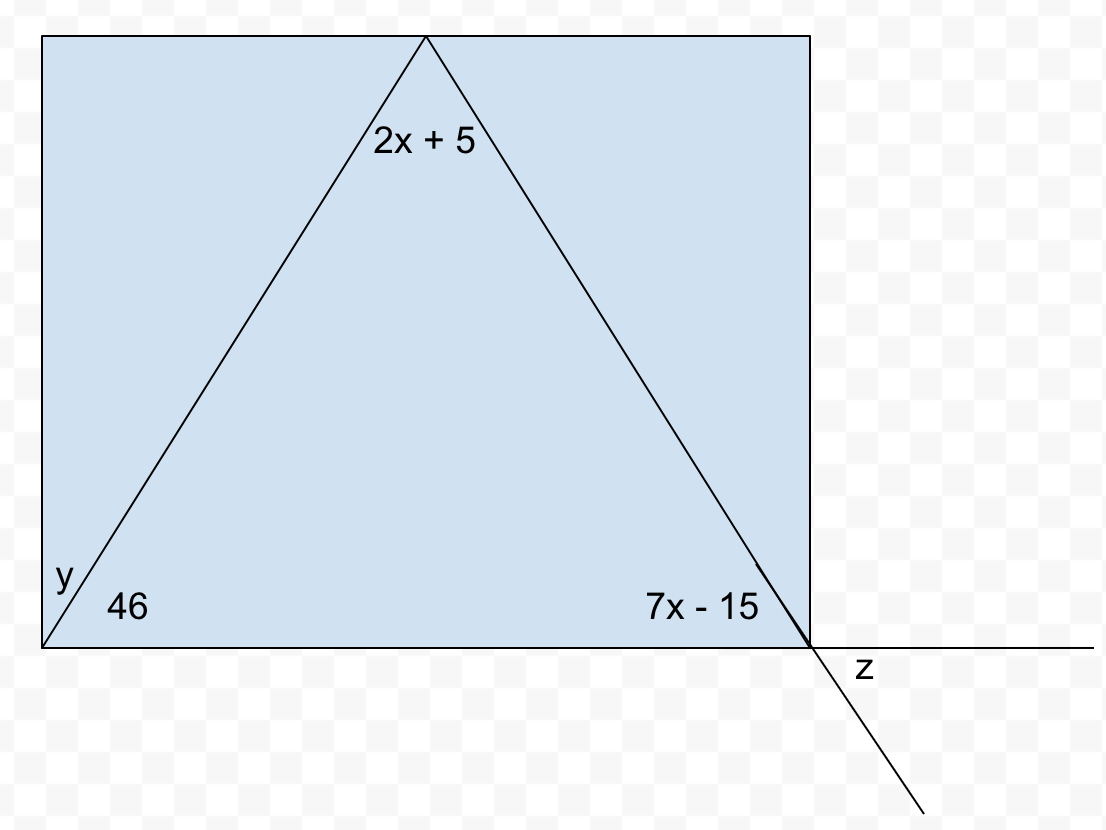Kindergarten
1st
2nd
3rd
4th
5th
6th
7th
8th
9th
10th
11th
12th
Higher Ed
Other
Subjects
ELA
Math
Science
Social Studies
Art
Computer Science
French
German
Music
Physical Education
Spanish
Other
Private Library
Angle Questions
starstarstarstarstarstarstarstarstarstar
by Courtney Paull
| 7 Questions
Note from the author:
Angle equations from a triangle, complementary and vertical angles1
1 pt
Find the value of x. (Just type a number)
2
1 pt
Find the value of the angle 2x + 5. (Just type a number)
3
1 pt
Find the value of the angle 7x - 15. (Just type a number)
4
1 pt
Find the value of the angle labeled y.
5
1 pt
Explain how you found the measurement of angle y.
6
1 pt
Find the value of the angle labeled z.
7
1 pt
Explain how you found the measurement of angle z.
Add to my formatives list